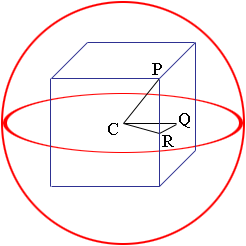Quandaries and Queries Who is asking: Student Level: All Question: A cube is inscribed inside a sphere with radius sqrt8cm. Find the (a) length of the cube (b) volume of the space inside the sphere but outside the cube. (Take Pi=3.14) Hi, I drew a diagram.C is the center of the sphere and the vertices of the cube are on the surface of the square. Suppose that the length of a side of the cube is s. Here are some facts that will help you answer the questions. |PC| = Sqrt(8) cm |PR| = |RQ| = s/2 Triangles PCR and CRQ arre both right triangles. Penny Go to Math Central